#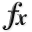N-D Test Functions N¶

class go_benchmark.NeedleEye(dimensions=2)

NeedleEye test objective function.

This class defines the Needle-Eye global optimization problem. This is a multimodal minimization problem defined as follows: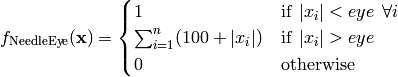Where, in this exercise,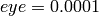.

Here,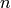represents the number of dimensions andfor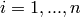.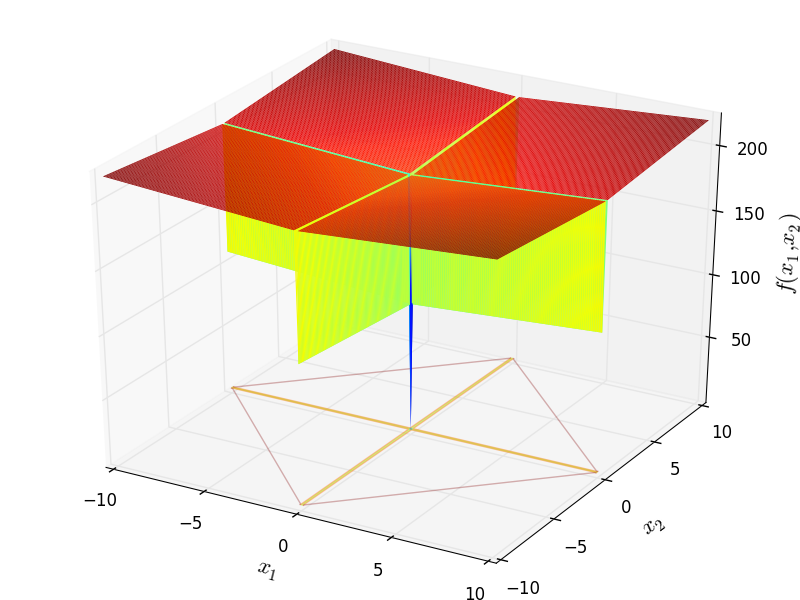Two-dimensional NeedleEye function

Global optimum: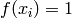for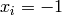forclass go_benchmark.NewFunction01(dimensions=2)

NewFunction01 test objective function.

This class defines the NewFunction01 global optimization problem. This is a multimodal minimization problem defined as follows: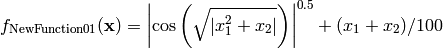Here,represents the number of dimensions andfor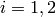.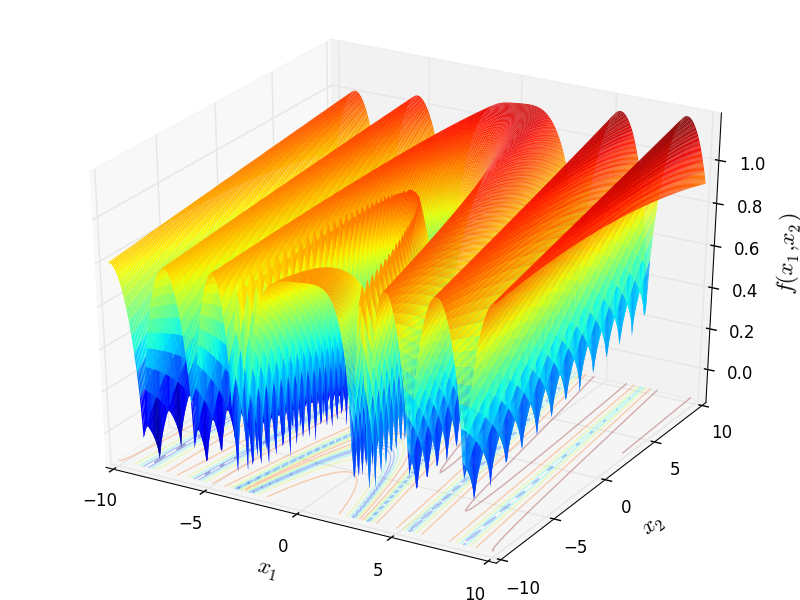Two-dimensional NewFunction01 function

Global optimum:for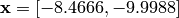class go_benchmark.NewFunction02(dimensions=2)

NewFunction02 test objective function.

This class defines the NewFunction02 global optimization problem. This is a multimodal minimization problem defined as follows:Here,represents the number of dimensions andfor.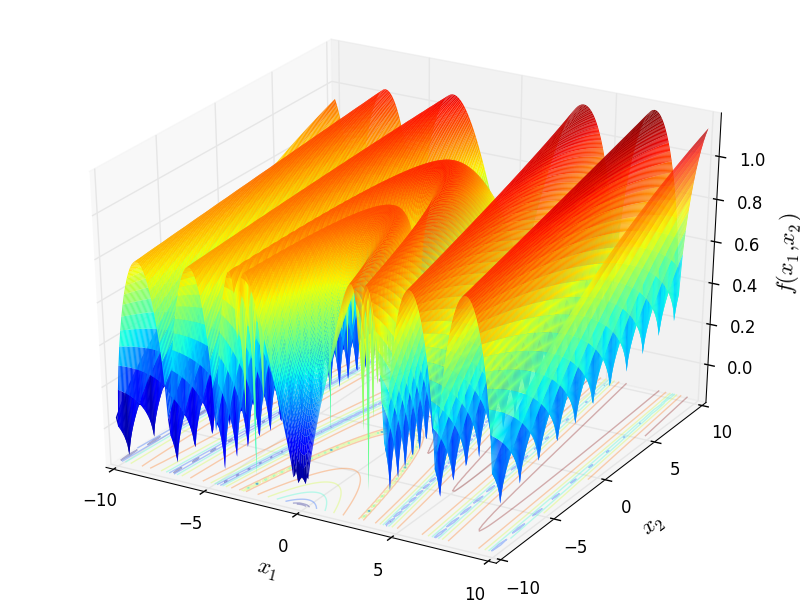Two-dimensional NewFunction02 function

Global optimum: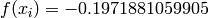for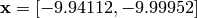class go_benchmark.NewFunction03(dimensions=2)

NewFunction03 test objective function.

This class defines the NewFunction03 global optimization problem. This is a multimodal minimization problem defined as follows: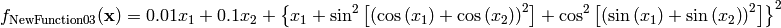Here,represents the number of dimensions andfor.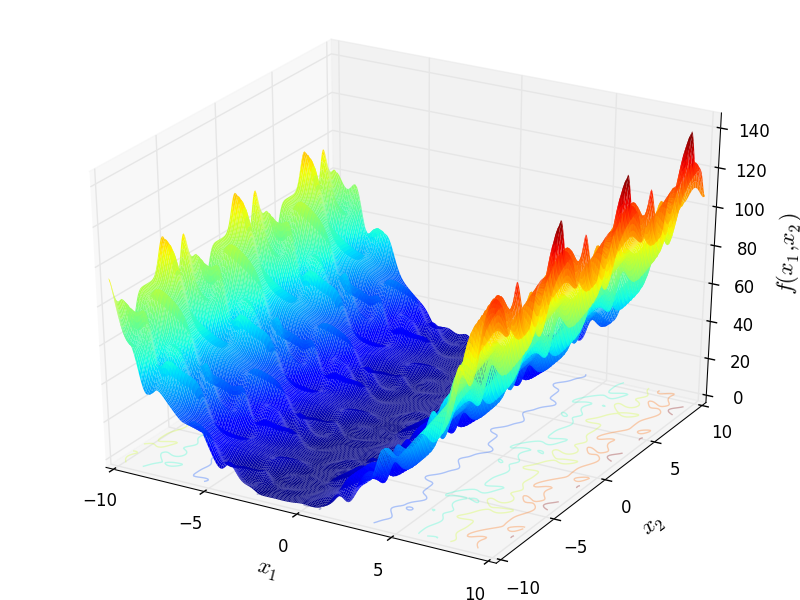Two-dimensional NewFunction03 function

Global optimum:for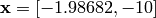#### Previous topic

N-D Test Functions M

#### Next topic

N-D Test Functions O